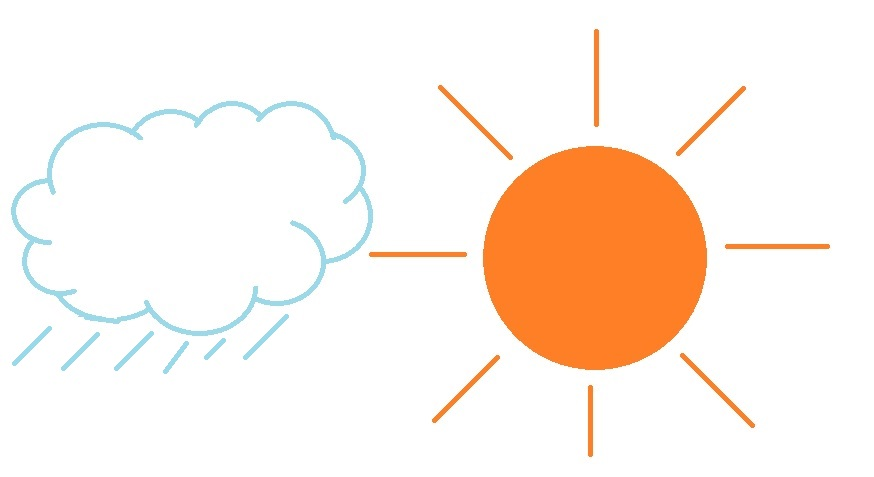Rain Or Shine

Probability Level 2The weather forecast stated that there would be 60% chance of rain on Saturday and 30% chance of rain on Sunday.

What is the probability (in percentage) of rain on at least one of these two days? (Assume the days are independent.)

×